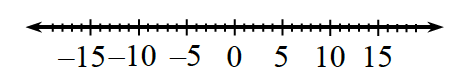### Home > CC1 > Chapter Ch4 > Lesson 4.1.3 > Problem4-38

4-38.

Copy the following problems, then use the number line to help you fill in $<$ (less than) or $>$ (greater than) on the blank line between each pair of numbers.1. $-4.84\text{ __ }-8.48$

• $-4.84$ is to the right of $-8.48$ on the number line.

$-4.84>-8.84$

1. $7 \text{ __ } - 7$

• $7$ is to the right of $-7$ on the number line. Also, positive numbers are always greater than negative numbers.

• $7>-7$

1. $-6.5\text{ __ }-5\frac{1}{2}$

• See part (a).

1. $-1\text{ __ }0$

• See part (b).